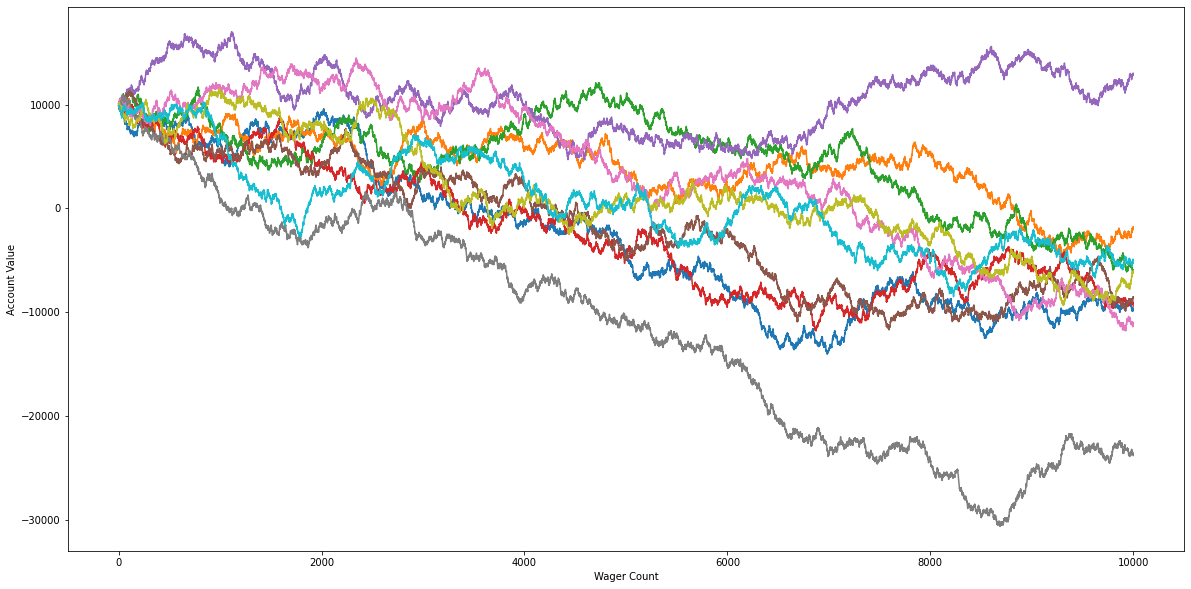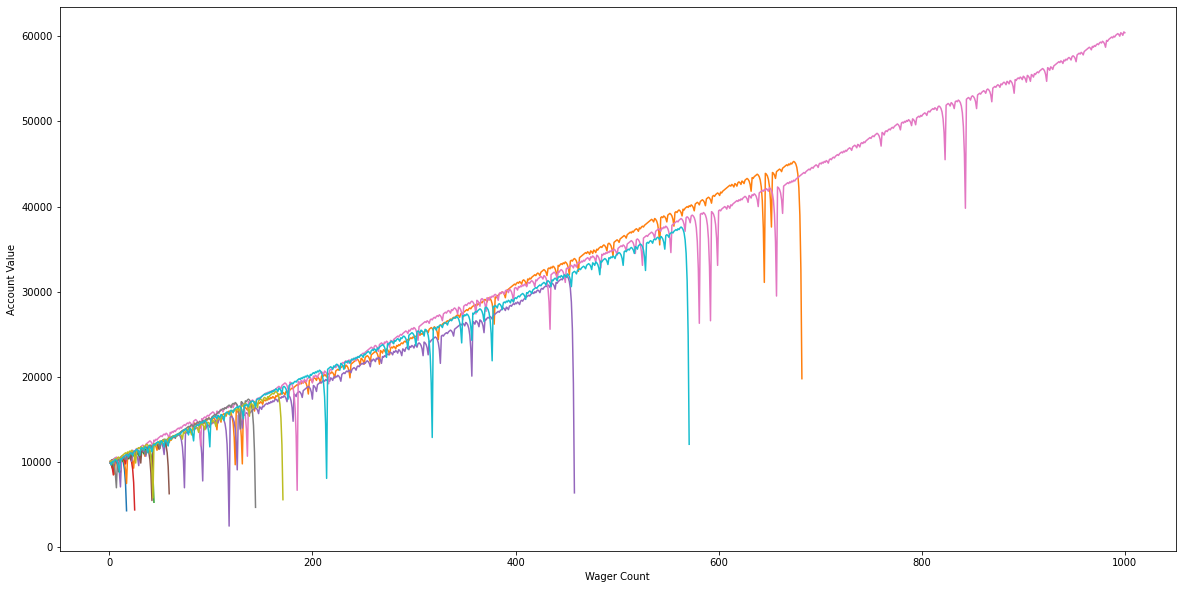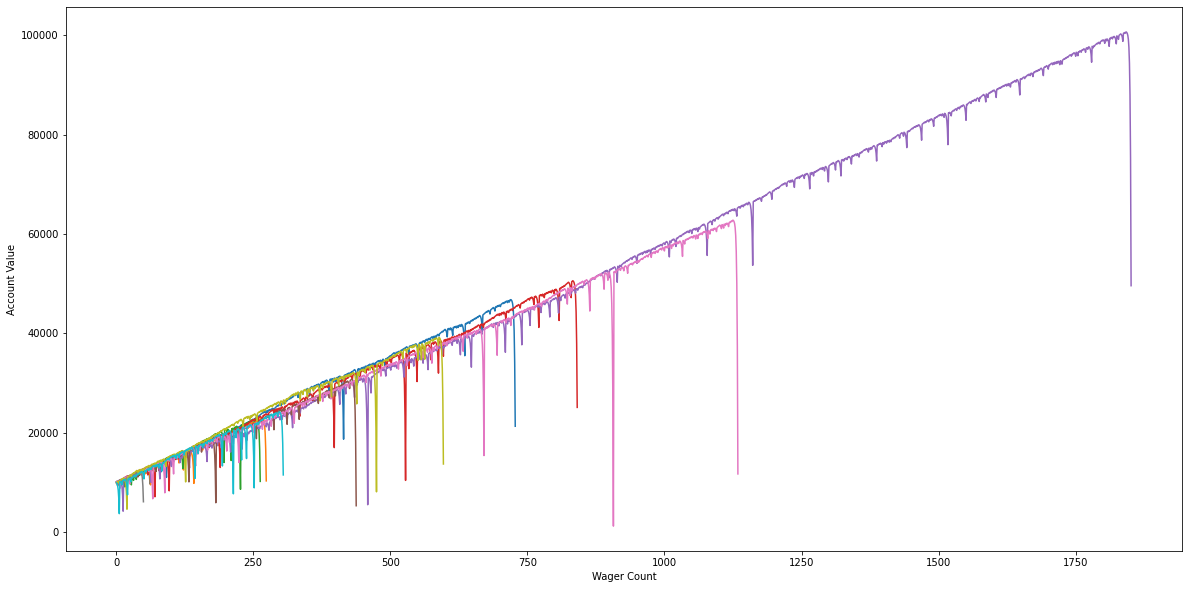# Monty Carlo Simulation with Python¶

A method to visualize outcomes and risks of any decision. Let's start with a simple gambling experiment.

1 - 50 house wins! 51 - 99 you win! - 100 house wins! Let's rolls a dice with 100 sides and see the effects of this.

In :
import random

In :
# Create a function which rolls the dice.
def rollDice():
roll = random.randint(1, 100)
if roll == 100:
return False
elif roll <= 50:
return False
elif 100 > roll > 50:
return True

In :
# Create a better (a person placing bets on the dice roll
def simple_bettor(funds, initial_wager, wager_count):
value = funds
wager = initial_wager

currentWager = 1

while currentWager <= wager_count:
if rollDice():
value += wager
else:
value -= wager
currentWager += 1

if value <= 0:
value = 'broke'

print('Funds: ', value)


In :
# Let's see some results while a 10 players play 10,000 games starting with $10,000 and betting$100 a game
number_of_bettors = 10
while number_of_bettors > 0:
simple_bettor(10000, 100, 10000)
number_of_bettors -= 1

Funds:  3600
Funds:  broke
Funds:  broke
Funds:  10600
Funds:  broke
Funds:  broke
Funds:  broke
Funds:  broke
Funds:  broke
Funds:  19200


Though many of our betters ended up broke we can map their progress throughout the games being played

In :
import matplotlib.pyplot as plt

In :
# Create a better (a person placing bets on the dice roll
def simple_bettor_plotter(funds, initial_wager, wager_count):
value = funds
wager = initial_wager

# For plotting purposes
wX = []
vY = []

currentWager = 1

while currentWager <= wager_count:
if rollDice():
value += wager
wX.append(currentWager)
vY.append(value)
else:
value -= wager
wX.append(currentWager)
vY.append(value)
currentWager += 1

if value <= 0:
value = 'broke'

plt.plot(wX, vY)

In :
# Let's plot 10 betters playing 10,000 games.
plt.figure(figsize=(20,10))
number_of_bettors = 10
while number_of_bettors > 0:
simple_bettor_plotter(10000, 100, 10000)
number_of_bettors -= 1
plt.ylabel('Account Value')
plt.xlabel('Wager Count')
plt.show()## Doubling on a loss strategy (Martingale Strategy)¶

In :
def doubler_bettor(funds, initial_wager, wager_count):
broke_count = 0
value = funds
wager = initial_wager

# For plotting purposes
wX = []
vY = []

currentWager = 1
previousWager = 'win'
previousWagerAmount = initial_wager

while currentWager <= wager_count:
if previousWager == 'win':
if rollDice():
value += wager
wX.append(currentWager)
vY.append(value)
else:
value -= wager
previousWager = 'loss'
previousWagerAmount = wager
wX.append(currentWager)
vY.append(value)
if value < 0:
broke_count += 1
break
elif previousWager == 'loss':
if rollDice():
wager = previousWagerAmount * 2
value += wager
wager = initial_wager
previousWager = 'win'
wX.append(currentWager)
vY.append(value)
else:
wager = previousWagerAmount * 2
value -= wager
if value < 0:
broke_count += 1
break
previousWager = 'loss'
previousWagerAmount = wager

wX.append(currentWager)
vY.append(value)

currentWager += 1
plt.plot(wX, vY)

In :
# Let's plot 10 betters playing 1000 games while double betting.
plt.figure(figsize=(20,10))
number_of_double_bettors = 10
while number_of_double_bettors > 0:
doubler_bettor(10000, 100, 1000)
number_of_double_bettors -= 1
plt.ylabel('Account Value')
plt.xlabel('Wager Count')
plt.show()The majority of people will go broke using the double or nothing strategy.

In :
# Let's plot 10 betters playing 10,000 games while double betting.
plt.figure(figsize=(20,10))
number_of_double_bettors = 10
while number_of_double_bettors > 0:
doubler_bettor(10000, 100, 10000)
number_of_double_bettors -= 1
plt.ylabel('Account Value')
plt.xlabel('Wager Count')
plt.show()When attempting to use the Martingale double strategy we can see that nobody get's anywhere near being able to complete 10,000 games. All go broke after <2000 games.

In [ ]: